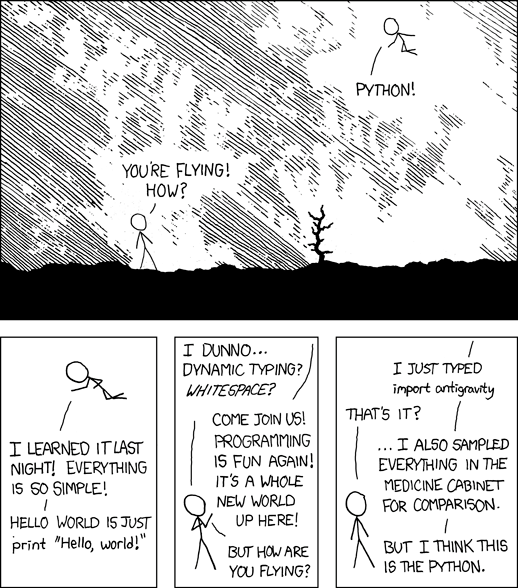# How to Retrieve the Year from a DateTime Object

## Problem Formulation and Solution Overview

This article will show you how to retrieve a year from a DateTime Object in Python. Whether that being a year from a DateTime Object, a DataFrame, or even a NumPy array.

💬 Question: How would we write code to retrieve the Year from a DateTime Object?

We can accomplish this task by one of the following options:

## Method 1: Use today().year()

This method uses the `today()` function to retrieve the current date and `year()` to extract the current year from that date.

```import datetime

cur_year = datetime.date.today().year
print(cur_year)```

The first line in the above code snippet imports Python’s built-in `datetime` library. This library allows access to and manipulation of dates and times in Python.

The following line first calls `datetime` (`cur_year = datetime`). This returns a `DateTime` class module similar to below:

Next, `date` is appended to `datetime` (`cur_year = datetime.date`) and returns a `datetime.date` class similar to below:

Then, `today()` is appended (`cur_year = datetime.date.today()`) and retrieves the current date.

Finally, `year()` is appended to retrieve the current year from the current date shown above. The result is output to the terminal.

## Method 2: Use now() and year()

This method uses the `now()` function to retrieve the current date and time and `year` to retrieve the current year from that date.

```import datetime

cur_year = datetime.datetime.now().year
print(cur_year)```

The first line in the above code snippet imports Python’s built-in `datetime` library. This library allows access to and manipulation of dates and times in Python.

The following line first calls `datetime` (`cur_year = datetime`). This returns a datetime class module similar to below:

Next, `datetime` is appended to `datetime `(`cur_year = datetime.datetime`) and returns a `datetime.date` class similar to below:

Then, `now()` is appended (`cur_year = datetime.date.now()`) and retrieves the current date and time.

Finally, `year` is appended to retrieve the current year from the current date and time shown above. The result is output to the terminal.

## Method 3: Use DatetimeIndex()

This method uses Panda’s `DatetimeIndex()` function to retrieve the `year` from each Date column in a DataFrame.

Before running this code snippet, the `Pandas` library needs to be installed. Click here for installation instructions.

```import pandas as pd

df['HIRE_YR'] = pd.DatetimeIndex(df['HIRE_DATE']).year
print(df['HIRE_YR'])```

The first line in the above code snippet imports the `Pandas` library. This library allows access to and manipulation of DataFrames.

The following line reads in two (2) columns and five (5) rows from the CSV file `staff.csv`. The results save to the DataFrame, `df`.

The following line retrieves the year from each record in the DataFrame and saves the results to a new column, by using `DatetimeIndex()` and passing it `df['HIRE_DATE']` as an argument. The results save to a new column `df['HIRE_YR']`.

The results are output to the terminal.

## Method 4: Use Timestamp()

This method uses the Panda’s `Timestamp()` function to retrieve the `year` from a Date column in a DataFrame.

Before running this code snippet, the `Pandas` library needs to be installed. Click here for installation instructions.

```import pandas as pd

the_date = pd.Timestamp(year=2022, month=12, day=25, hour=1, second=1, tz='US/Pacific')
print(the_date.year)```

The first line in the above code snippet imports the `Pandas` library. This library allows access to and manipulation of DataFrames.

The following line calls the `timestamp()` function and passes it the following arguments:

• A year
• A month
• A Day
• An Hour
• A Second
• A TimeZone

The results save to `the_date`. If output to the terminal, the following would display:

Finally, the `year` is retrieved and output to the terminal.

## Method 5: Use strptime() and a lambda

This method uses the `datetime` and `strptime()` function in conjunction with a `lambda` to retrieve the `year` from a Date column in a DataFrame.

Before running this code snippet, the `Pandas` library needs to be installed. Click here for installation instructions.

```import datetime
import pandas as pd

df.loc[:,'HIRE_YEAR'] = df.loc[:,'HIRE_DATE'].map(lambda x: datetime.datetime.strptime(str(x),'%d-%b-%y').year)
print(df)```

The first two (2) lines of the above snippet import the `datetime` library and the `Pandas` library. These two (2) libraries allow access to and manipulation of datetimes as well as DataFrames.

The following line reads in two (2) columns and five (5) rows from the CSV file `staff.csv`. The results save to the DataFrame, `df`.

The following line uses `loc`, `map()`, a `lambda`, `strptime()`, and `year` to retrieve the year from each row in the `df['HIRE_DATE']` column of the DataFrame. The results save as a new column, `df['HIRE_YEAR']`, in the DataFrame.

## Method 6: Use to_datetime()

Thie method uses the NumPy and Pandas library in conunction with `to_datetime()` to retrieve the `year`.

Before running this code snippet, the NumPy and `Pandas` libraries must be installed.

```import numpy as np
import pandas as pd

dates = np.array(['2010-10-17', '2011-05-13', '2012-01-15'], dtype=np.datetime64)
print(pd.to_datetime(dates).year)```

The first two lines of the above code snippet import the NumPy and the `Pandas` library.

The following line is passed a NumPy array containing three (3) dates. These dates are set to the type of `datetime64`. The results save to dates. If output to the terminal, the following would display”

Finally, the year is retrieved from the above and output to the terminal.

## Bonus:

If you have a list of dates and the location of the year falls in the same place, slicing works!

```dates = ['2021-01-15', '2022-08-01', '2023-09-01', '2224-12-01']
results = [d[:4] for d in dates]
print(results)```

Results

## Summary

This article has provided 7 ways to retrieve a year from a DateTime object to select the best fit for your coding requirements.

Good Luck & Happy Coding!

## Programming Humor – Python“I wrote 20 short programs in Python yesterday. It was wonderful. Perl, I’m leaving you.” — xkcd